Courses

# Spillways and Energy Dissipators (Part - 4) Civil Engineering (CE) Notes | EduRev

## Civil Engineering (CE) : Spillways and Energy Dissipators (Part - 4) Civil Engineering (CE) Notes | EduRev

The document Spillways and Energy Dissipators (Part - 4) Civil Engineering (CE) Notes | EduRev is a part of the Civil Engineering (CE) Course Water Resources Engineering.
All you need of Civil Engineering (CE) at this link: Civil Engineering (CE)

Discharge characteristics of ogee crests-uncontrolled flow

For an ogee crested control weir for a spillway without any control with a gate, the free flow discharge equation is given as

Q =CLe He3/2            (3)

Where Q is the discharge (in m3/s), Cd is the coefficient of discharge, Le is the effective length of crest (in m), including velocity of approach head. The discharge coefficient, Cd, is influenced by a number of factors, such as:

1. Depth of approach
2. Relation of the actual crest shape to the ideal nappe shape
3. Upstream face slope
4. Downstream a pron interference, and
5. Downstream s ubmergence

The effect of the above mentioned factors on the variation of discharge and calculation for effective length are mentioned in the following paragraphs.

1. Effect of  depth of approach

For a high sharp-crested ogee shaped weir, as that of a Overfall spillway of a large dam, the velocity of approach is small and the lower nappe flowing over the weir attains maximum vertical contraction. As the approach depth is decreased, the velocity of approach increases and the vertical contraction diminishes. For sharp-crested weirs whose heights are not less than about one-fifth of the head producing the flow, the coefficient of discharge remains fairly constant with a value of about 1.82 although the contraction diminishes. For weir heights less than about one-fifth the head, the contraction of the flow becomes increasingly suppressed and the crest coefficient decreases. This is the case of an ogee crested chute spillway control section. When the weir height becomes zero, the contraction is entirely suppressed and the weir turns into a broad crested one, for which the theoretical coefficient of discharge is 1.70. The relationship of the ogee crest coefficient of discharge Cd  for various values of P/Hd  where P is the height of the weir above base and Hd is the design head, is given in Figure 25. The coefficients are valid only when ogee is formed to the ideal nappe shape.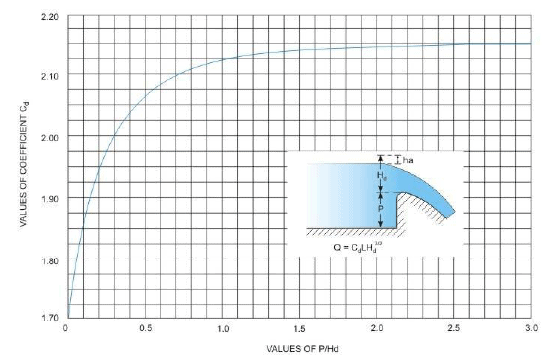FIGURE 25. Coefficient of discharge (Cd) variation due to height of a vertical faced ogee crest

2. Effect of the crest shape differing from the ideal nappe shape

When the ogee crest is formed to a shape differing from the ideal nappe shape or when the crest has been shaped for a head larger or smaller than the one under consideration, the coefficient of discharge will differ from that given in the previous section. A wider crest shape will reduce the coefficient of discharge while a narrower Crest Shape will reduce the coefficient. The application of this concept is required to deduce the discharge flowing over a spillway when the flow is less or more than the design discharge. The variation of the coefficient of discharge in relation to H/Hd, where H is the actual head and His the design head, is shown in Figure 26.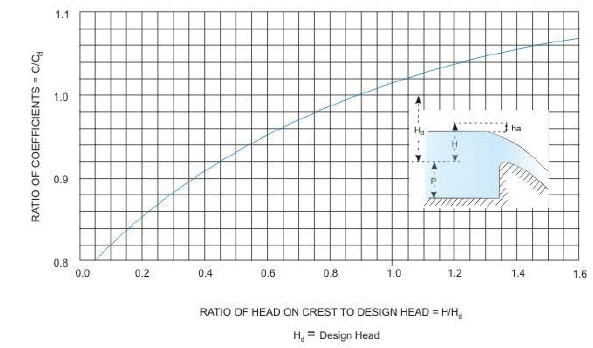FIGURE 26. Coefficient of discharge other than the design head

3. Effect of upstream face slope

For small ratio of P/Hd where P is the height of the weir and Hd the design head, as for the approach to a chute spillway, increase of the slope of upstream face tends to increase the coefficient of discharge, as shown in Figure 27. This figure shows the ratio of the coefficient for ogee crest with a sloping face to that with vertical face. For large ratios of P/Hd, the effect is a decrease of the coefficient. The coefficient of discharge is reduced for large ratios P/Hd only for relatively flat upstream slopes.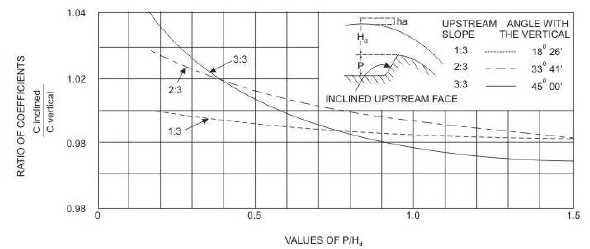Figure 27. Coefficient of discharge variation with upstream face inclination.

4. Effect of downstream apron interference and downstream submergence

This condition is possible for dams of relatively small heights compared to the natural depth of the river, when the water level downstream of the weir crest is high enough to affect the discharge, the condition being termed as submerged. The conditions that after the coefficient of discharge in this case are the vertical distance from the crest of the over flow to the downstream apron and the depth of flow in the downstream channel, measured above the apron.

Five distinct characteristic flow conditions can occur below an overflow crest, depending on the relative positions of the apron and the downstream water surface:

• The flow will continue at supercritical stage
• A partial or incomplete hydraulic jump will occur immediately downstream from the crest
• A true hydraulic jump will occur
• A drowned jump will occur in which the high-velocity jet will follow the face of the overflow and then continue in an erratic and fluctuating path for a considerable distance under and through the slower water, and E. No jump will occur - the jet will break away from the face of the overflow and ride along the surface for a short distance and then erratically intermingle with the slow moving water underneath.

According to USBR (1987), the relationship of the floor positions and downstream submergences which produce these distinctive flows can be shown in a graph as in Figure 28.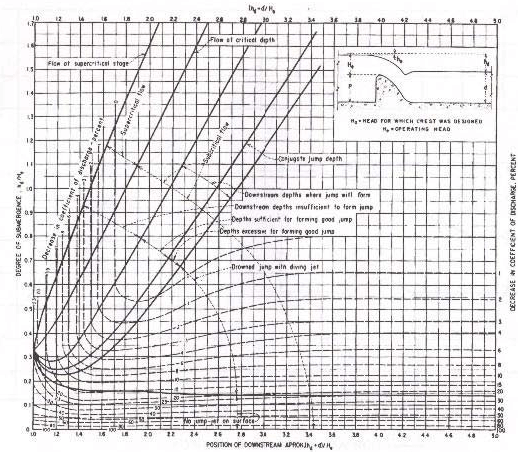FIGURE 28. Effects of downstream influences on flow over weir crests

Usually for large dams the cases A,B or C with dominate and the decrease in the coefficient of discharge is due principally to the back pressure effect of the downstream apron and is independent of any submergence effect due to tail water. Cases D and E can be expected t o be found in low-height dams like small height diversion or navigation dam. Figure 29, adapted from USBR (1987), shows the effect of downstream apron conditions on the coefficient of discharge. It may be noted that this curve plots the same data represented by the vertical dashed lines of Figure 28 in a slightly different form. As the downstream apron level nears the crest of the overflow (h+ d / He) approaches 1.0), where hd is the difference of total energy on upstream and the water level downstream, d is the downstream water depth and He is the total energy upstream measured above the crest of the weir, the coefficient of discharge is about 77 percent of that for un-retarded flow. From Figure 29 it can be seen that when the ratio of  (h+ d / He) values exceed about 1.7, the downstream floor position has little effect on the coefficient, but there is a decrease in the coefficient caused by tail water submergence. Figure 30 shows the ratios of the coefficient of discharge where affected by tailwater conditions, to that coefficient for free flow conditions. This curve plots the data represented by the horizontal dashed lines on Figure 28 in a slightly different form. Where the dashed lines of Figure 28 are curved, the decrease in the coefficient is the result of a combination of tail-water effects and downstream apron position.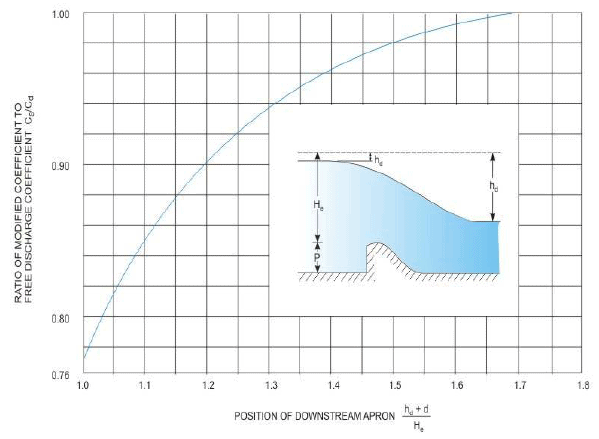FIGURE 29. Ratio of discharge coefficients resulting from the effect of the apron on flow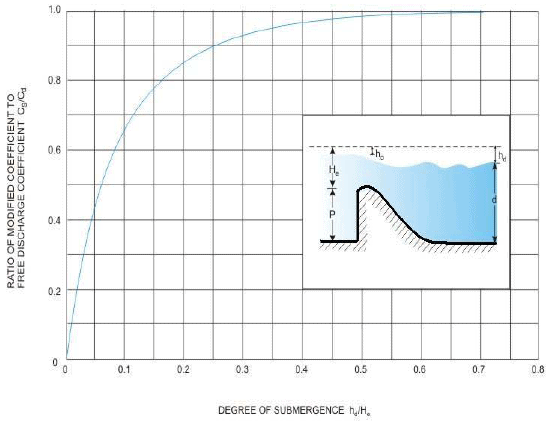FIGURE 30. Ratio of discharge coefficients caused by tailwater effects

If the ordinate of Figure 30 is changed from hd/He  to 1 - hd/He , that is, equal to He - hd/ He = h/He where h is the downstream water depth measured above crest, then the curve of Figure 30 may be transposed as in Figure 31.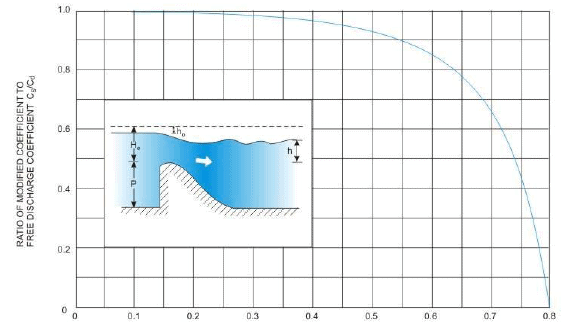Figure 31. Ratio of submerged discharge coefficient to that without submergence effect This figure is similar to that given in Figure 30. but with different ordinate value

The total head on the crest He, does not include allownces for approach channel friction losses due to curvature into the inlet section, and inlet or transition losses. Where the design of the approach channel results in appreciable losses, they must be added to He to determine reservoir elevations corresponding to the discharges given by the discharge equation.

Where the crest piers and abutments are shaped to cause side contractions of the overflow, the effective length, Le, will be the net length of the crest, L. The effect of the end contractions may be taken into account by reducing the net length of crest as given below:

Le = L - 2(N�Kp + Kα)�He

Where L, Le and H have been explained before, N is the number of piers and Kand Ka are the pier and abutment contraction coefficients. The reason for the reduction of the net length may be appreciated from Figure 32.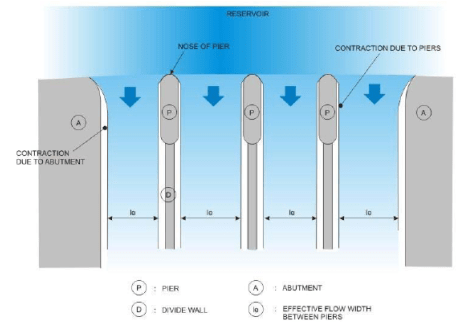Figure 32. Abutment and pier contractions shown on a spillway plan

The pier contraction coefficient Kp depends upon the following factors:

1. Shape and location of the pier nose
2. Thickness of the pier
4. Approach velocity

For the condition of flow at the design head, the average values of pier contraction coefficients may be assumed as shown in Figure 33.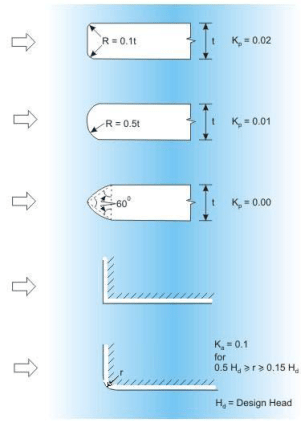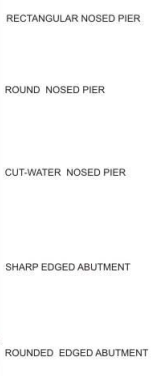Figure 33. Recommended values of Kc and K,

The abutment contraction coefficient is seen to depend upon the following factors:

1. Shape of abutment
2. Angle between upstream approach wall and the axis of flow
4. Approach velocity

For the condition of flow at the design head, the average value of abutment contraction coefficients may be assumed as shown in Figure 33.

For flow at head other than design head, the values of Kp and Ka may be obtained from graphical plots given in IS: 6934-1973 “Recommendations for hydraulic design of high ogee overflow spillways”.

Discharge characteristics of ogee crests-controlled spillway

The discharge for gated crests at partial gate opening is similar to flow through a lowhead orifice and may be computed by the following equation recommended by the Bureau of Indian Standards code IS:6934-1998 “Hydraulic design of high ogee overflow spillways-recommendations”.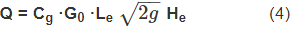Where Q is the discharge (in m3/s), Cg is the gated coefficient of discharge, G0 is the gate opening (in m), Lis the effective length of crest, g is the acceleration due to gravity, and He is the hydraulic head measured from the centre of the orifice (in m).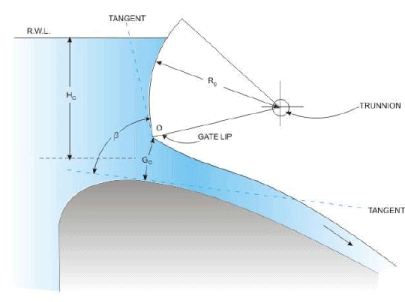Figure 34. Partially opened radial gate discharging flow

Usually for high head spillways, radial gates are common and Figure 34 shows the position of a partially opened radial gate over an ogee-crested spillway. The gate opening G0 may be seen to be measured as the shortest distance from the gate lip to the ogee crest profile meeting at G. The angle β is seen to be measured between the tangent at G and the tangent of the radial gate at gate lip. Figure 35 presents a curve relating the coefficient of gated discharge Cg with the angle β.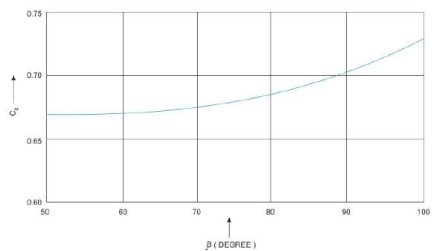Figure 35. Coefficient of gated discharge Cg variation with lip angle β

The curve presents an average value of Cg determined for various approach and downstream conditions and may be used for preliminary design purposes. In fact, it may be noticed that the discharge equation mentioned above for calculating flow through a gated spillway as recommended in IS: 6934-1998 may not be strictly correct as the gate opening becomes larger, comparable to the hydraulic head Hc

Offer running on EduRev: Apply code STAYHOME200 to get INR 200 off on our premium plan EduRev Infinity!

156 docs|6 tests

,

,

,

,

,

,

,

,

,

,

,

,

,

,

,

,

,

,

,

,

,

;